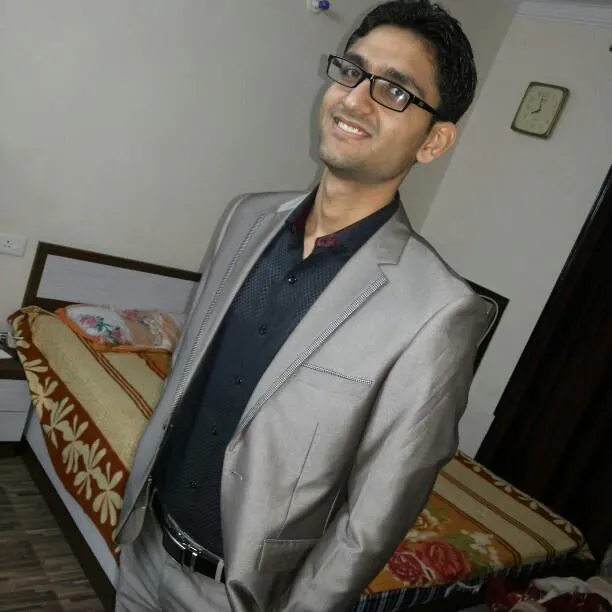Published © LGPL

# electricity meter reading via SMS

Usually in india a person has to go and check the meter reading after every three months and it is quite a hectic and long process.

IntermediateFull instructions provided1,345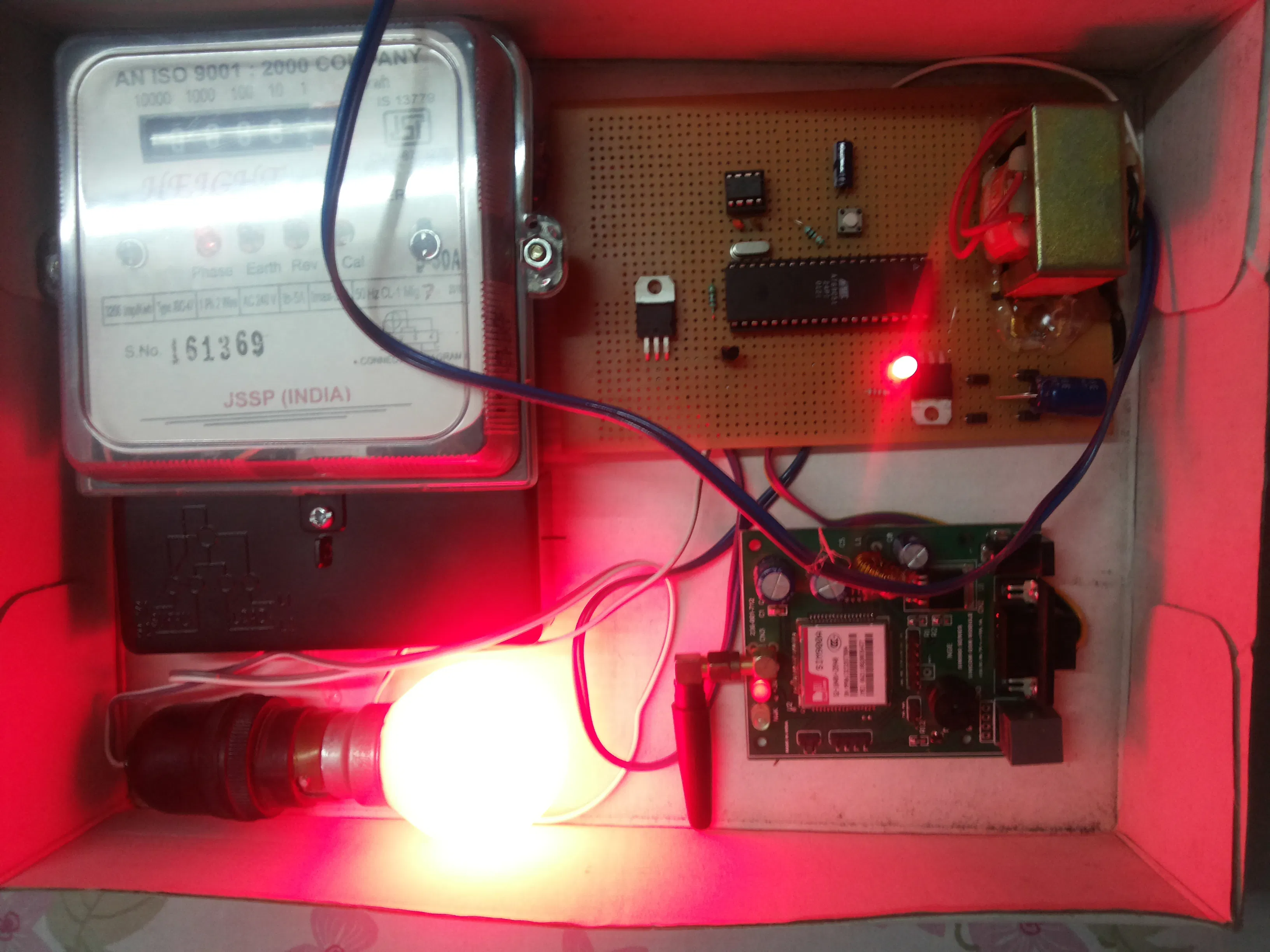## Things used in this project

### Hardware components

 electricity meter
×1
 GSM modem
×1
 AT89C51 ic
×1
 33 PF CAPACITOR
×2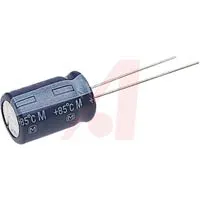Capacitor 10 µF
×1
 CRYSTAL 11.0592MHZ
×1
 TECTILE SWITCH
×1
 10 K RESISTANCE
×1
 24C04 ic
×1
 8 PIN IC BASE
×1
 TRANSISTOR BC547
×1
 TRANSFORMER 12-0-12 V
×1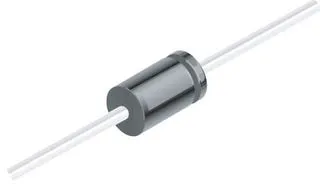1N4007 – High Voltage, High Current Rated Diode
×4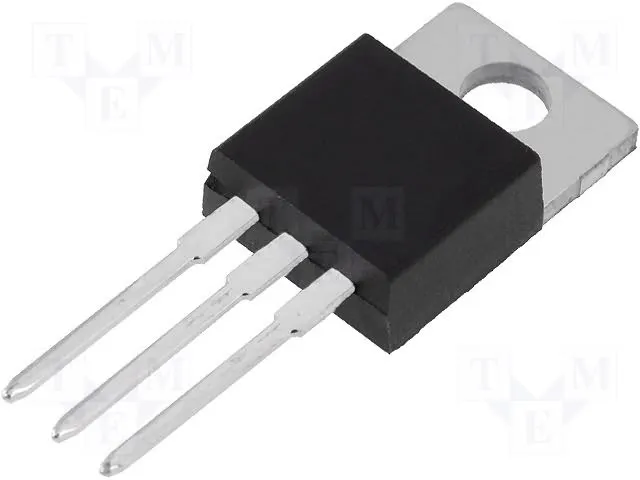Linear Regulator (7805)
×1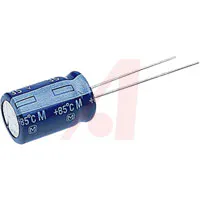Capacitor 1000 µF
×1

### Hand tools and fabrication machinesSoldering iron (generic)

Read more

## Schematics

### circuit diagram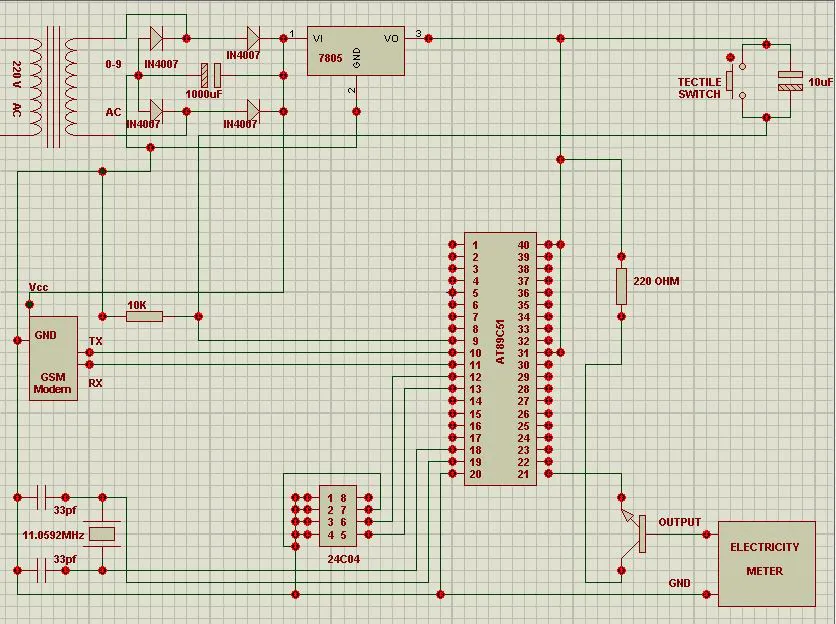## Code

### project code

C/C++
```#include<reg51.h>
#include<intrins.h>

sbit meter=P2^0;

sbit SCL=P3^2;
sbit SDA=P3^3;

void delay(unsigned int w)
{
unsigned int q;
for(q=0;q<w;q++);
}

void start()
{
SDA = 1 ;	   _nop_() , _nop_() , _nop_() ;
SCL = 1 ;	   _nop_() , _nop_() , _nop_() ;
SDA = 0 ; 	   _nop_() , _nop_() , _nop_() ;
SCL = 0 ;	   _nop_() , _nop_() , _nop_() ;
}

void stop()
{
SDA = 0 ;	 _nop_() , _nop_() , _nop_() ;
SCL = 1 ;	 _nop_() , _nop_() , _nop_() ;
SDA = 1 ;	 _nop_() , _nop_() , _nop_() ;
SCL = 0 ;	 _nop_() , _nop_() , _nop_() ;
}

void clock()
{
SCL = 1 ;	 _nop_() ; _nop_() ; _nop_() ;
SCL = 0 ;	 _nop_() ; _nop_() ; _nop_() ;
}

void nack()
{
SDA = 1 ;	  clock() ;
}

void waitack()
{
SDA = 1 ;	  clock() ;
}

void opdat(unsigned char dat)
{
unsigned char i ;
for ( i = 0 ; i < 8 ; i++ )
{
if ( ( dat >> ( 7 - i ) ) & 0x01 ) 	SDA = 1 ;
else	 			SDA = 0 ;
clock() ;
}
}

void i2c_write(unsigned char dat,unsigned char addr)
{
start();		 //dummy write start
opdat(0xa0);	//send dev addr
waitack();
opdat(addr);
waitack();
opdat(dat);		//write data
waitack();
stop();
}

unsigned char getdat()
{
unsigned char i , dat;
SDA = 1;	   _nop_() , _nop_() , _nop_();
for ( i = 0 ; i < 8 ; i++ )
{
dat = ( ( ( dat << 1 ) & 0xfe ) | SDA );
clock();
}
return dat;
}

unsigned char i2c_read(unsigned char addr)
{
unsigned char rdata;
start();		//dummy write start
opdat(0xa0);	//send dev addr
waitack();
opdat(addr);
waitack();
start();				//start read
opdat(0xa1);   //give device adddr or with for read
waitack();	//operation coz lsb gives r|w
rdata = getdat();
nack() ;
stop() ;
return(rdata);
}

void tx(unsigned char num)
{
SBUF = num;
while(TI == 0);
TI = 0;
}

void tx_str(unsigned char *y)
{
while(*y!='\0')
{
tx(*y);
y++;
}
}

void main()
{
unsigned int e;
unsigned char x,r,q,w,t,y,u,i,arr1,arr,arr2;

SCON=0x50;
TMOD=0x20;
TH1=0xfd;
TR1=1;

delay(60000);
delay(60000);
delay(60000);
delay(60000);
delay(60000);
delay(60000);
delay(60000);
delay(60000);
delay(60000);
delay(60000);
delay(60000);
delay(60000);
delay(60000);
qwe:
tx_str("AT");
tx(0x0d);
delay(60000);
tx_str("AT+CMGF=1");
tx(0x0d);
delay(60000);
delay(60000);

while(1)
{
if(meter==1)
{
x=i2c_read(7);
delay(100);
if(x<9)
{
i2c_write((x+1),7);
delay(12000);
}
else
{
i2c_write(0,7);
delay(1200);
w=i2c_read(6);
delay(100);
if(w<9)
{
i2c_write((w+1),6);
delay(12000);
}
else
{
i2c_write(0,6);
delay(1200);
q=i2c_read(5);
delay(100);
if(q<9)
{
i2c_write((q+1),5);
delay(12000);
}
else
{
i2c_write(0,5);
delay(1200);
r=i2c_read(4);
delay(100);
if(r<9)
{
i2c_write((r+1),4);
delay(12000);
}
else
{
i2c_write(0,4);
delay(1200);
t=i2c_read(3);
delay(100);
if(t<9)
{
i2c_write((t+1),3);
delay(12000);
}
else
{
i2c_write(0,3);
delay(1200);
y=i2c_read(2);
delay(100);
if(y<9)
{
i2c_write((y+1),2);
delay(12000);
}
else
{
i2c_write(0,2);
delay(1200);
u=i2c_read(1);
delay(100);
if(u<9)
{
i2c_write((u+1),1);
delay(12000);
}
else
{
i2c_write(0,1);
delay(1200);
i=i2c_read(0);
delay(100);
if(i<9)
{
i2c_write((i+1),0);
delay(12000);
}
}
}
}
}
}
}
}
}
if(RI==1)
{
w=SBUF;
RI=0;
if(w=='+')
{
e=0;
while(RI==0)
{
e=e+1;
if(e>30000)
goto qwe;
}
RI=0;
q=SBUF;
e=0;
while(RI==0)
{
e=e+1;
if(e>30000)
goto qwe;
}
RI=0;
q=SBUF;
e=0;
while(RI==0)
{
e=e+1;
if(e>30000)
goto qwe;
}
RI=0;
q=SBUF;
if(q=='T')
{
delay(30000);
tx_str("AT+CMGR=1");
tx(0x0d);
delay(60000);
zxc:
e=0;
while(RI==0)
{
e=e+1;
if(e>30000)
{
goto qwe;
}
}
RI=0;
r=SBUF;
if(r!=':')
goto zxc;
r=0;
xcv:
e=0;
while(RI==0)
{
e=e+1;
if(e>30000)
{
goto qwe;
}
}
RI=0;
r=SBUF;
if(r!='+')
goto xcv;
r=0;
asd:
e=0;
while(RI==0)
{
e=e+1;
if(e>30000)
{
goto qwe;
}
}
RI=0;
arr2[r]=SBUF;
r=r+1;
if(SBUF!='"')
goto asd;
r=0;
wer:
e=0;
while(RI==0)
{
e=e+1;
if(e>30000)
{
goto qwe;
}
}
RI=0;
r=SBUF;
if(r!='*')
goto wer;
r=0;
ert:
e=0;
while(RI==0)
{
e=e+1;
if(e>30000)
{
goto qwe;
}
}
RI=0;
arr[r]=SBUF;
r=r+1;
if(SBUF!='#')
goto ert;
if(r==11)
{
if(arr=='R' && arr=='E' && arr=='A' && arr=='D' && arr=='I' && arr=='N' && arr=='G' && arr==' ' && arr=='1' && arr=='1')
{
for(t=0;t<8;t++)
{
arr1[t]=i2c_read(t);
delay(100);
}
tx_str("AT+CMGS=");
tx('"');
tx('0');
for(t=2;t<12;t++)
{
tx(arr2[t]);
}
tx('"');
tx(0x0d);
delay(20000);
tx_str("VALUE - ");
for(t=0;t<8;t++)
{
tx(0x30+arr1[t]);
if(t==4)
tx('.');
}
tx(0x1a);
delay(60000);
delay(60000);
delay(60000);
delay(60000);
goto qwe;
}
}
}
goto qwe;
}
}
}
}
```

## Credits

### Sumit Grover

8 projects • 24 followers